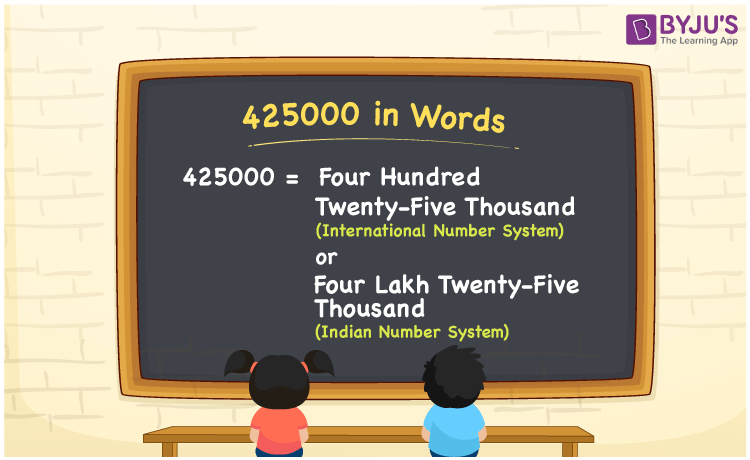# 425000 in Words

According to the International Number System, the number 425000 in words is “Four hundred twenty-five thousand”. Whereas in the Indian Number System, 425000 is expressed as “Four lakh twenty-five thousand”. Go through the article below to learn the method of writing the number 425000 in words using the place value system in detail.

 425000 in Words (International Number System): Four Hundred Twenty-five Thousand. 425000 in Words (Indian Number System): Four Lakh Twenty-five Thousand Four hundred twenty-five thousand in Numerical Form: 425000.

## 425000 in English Words## How to Write 425000 in Words?

The place value table for the number 425000 is provided below.

 Lakhs Ten-thousands Thousands Hundreds Tens Ones 4 2 5 0 0 0

The expanded form of 425000 is as follows:

= 4 × Lakh + 2 × Ten thousand + 5 × Thousand + 0 × Hundred + 0 × Ten + 0 × One

= 4 × 100000 + 2 × 10000 + 5 × 1000 + 0 × 100 + 0 × 10 + 0 × 1

= 400000 + 20000 + 5000

= 425000

= four hundred twenty-five thousand

Hence, 425000 in words is four hundred twenty-five thousand.

425000 in words – Four hundred twenty-five thousand

Is 425000 an odd number? – No

Is 425000 an even number? – Yes

Is 425000 a perfect square number? – No

Is 425000 a perfect cube number? – No

Is 425000 a prime number? – No

Is 425000 a composite number? – Yes

## Frequently Asked Questions on 425000 in Words

Q1

### How to write 425000 in words as per the International Number System?

According to the International Number System, 425000 in words is four hundred twenty-five thousand.

Q2

### Simplify 400000 + 25000, and express it in words.

Simplifying 400000 + 25000, we get 425000. Hence, 425000 in words is four hundred twenty-five thousand.

Q3

### How to write 425000 in words as per the Indian Number System?

According to the Indian Number System, 425000 in words is four lakh twenty-five thousand.